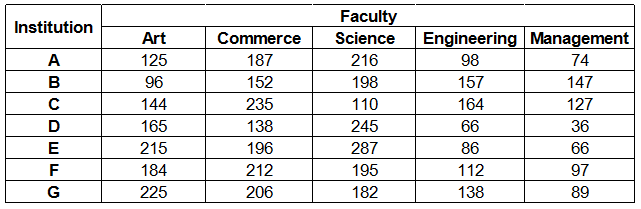### IBPS Clerk 2018 Question 19

Instructions

Study the following table carefully and answer the questions given below :
Number of students studying in different faculties in Seven institutionsQuestion 19

# What is the percentage of students studying Engineering in institute C to the total students of all institutions studying Engineering ? (rounded to the nearest integer)

Solution

Total students studying Engineering in institute C = 164

Total students of all institutions studying Engineering

= 98 + 157 + 164 + 66 + 86 + 112 + 138 = 821

=> Required % = $$\frac{164}{821} \times 100 = 19.97 \%$$

$$\approx 20 \%$$